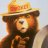# Convert TSW to thinkorswim

N

#### necktoppc

##### New member
Hi, can someone convert this tsw to tos? Thank you!
Code:
``````Use @ES.D or @MES.D 5 minute bars, with Exchange time for the chart.
//based on Art Collins webinar
//modifications by Kevin J. Davey
//
//
// [email protected]
//
//Development completed June 30, 2019
//
//
//non symmetrical exit (OK for stock indices)
{Chart Info:
Symbol#1 @MES.D
Bar Length 5 min
Start Date 6/30/2006
End Date present
Session regular
Strategy Name:
Strategy - Properties For All:
General Tab
Commission (per Share/Contract) .64
Position Slippage (per Share/Contract) 1.25
Back Testing Resolution check/not checked not checked, 1 min bars
Details if checked
Max Number of bars study will reference 50
Position limits checked/not checked not checked
Details if checked
Backtesting Tab
"Fill Entire Order when trade price exceeds limit price" must be chosen
Walkforward Optimization:
Total Iterations:35
Fitness Function: net profit
Anchored/Unanchored: unanchored
Parameters, Ranges:
Stopl=1000-2500,250
}
variables:
stopL ( 0 ) ,
pf ( 0 ) ;
if date >= 1140107 and date < 1150107 then
begin
stopL = 40 ;
end ;
if date >= 1150107 and date < 1160107 then
begin
stopL = 40 ;
end ;
if date >= 1160107 and date < 1170106 then
begin
stopL = 35 ;
end ;
if date >= 1170106 and date < 1180108 then
begin
stopL = 35 ;
end ;
if date >= 1180108 and date < 1190109 then
begin
stopL = 40 ;
end ;
if date >= 1190109 and date < 1200109 then
begin
stopL = 40 ;
end ;
if date >= 1200109 and date < 1210109 then
begin
stopL = 25 ;
end ;

var: openp(2);
if date >= 1140107 THEN begin
If time=835 then openp=open;
If time=840 then begin
If low>openp and (RSI(close,5)<50 or (c>c and c>c)) then begin
end;

If high<openp and (RSI(close,5)>50 or (c<c and c<c) ) then begin
sellshort next bar at market;
end;

end;
If time>=1100 and openpositionprofit>0 then beginHow do I convert this ATR% chart study into a scanner watchlist column? Questions 5